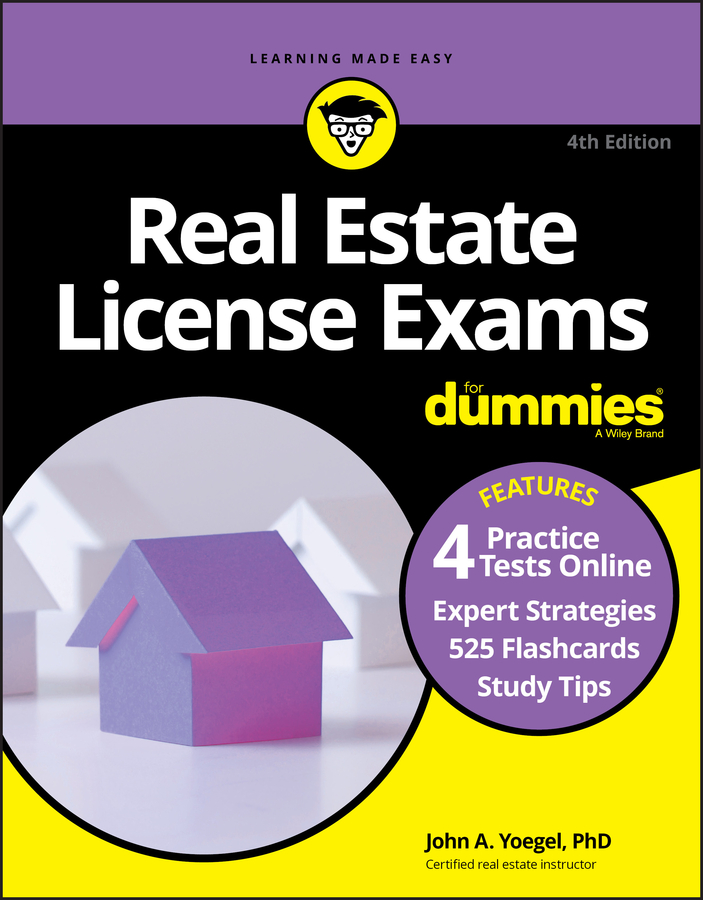##### Real Estate License Exams For Dummies with Online Practice TestsAlthough you may see a few questions on the Real Estate License Exam about how taxes work, the math question you’re most likely to see on your state licensing exam is one in which you must calculate the taxes on a piece of property. The basic mathematical procedure is to multiply the assessed value by the tax rate.

Assessed value of the property x tax rate = taxes due

Because different municipalities in your state may use the three different methods, here are three problems using mills per dollar of assessed value, dollars of tax per hundred dollars of assessed value, and dollars of tax per thousand dollars of assessed value. You need to be able to calculate taxes using all three methods.

Take note that the tax rate in the examples that follow stays the same.

## When the tax rate is expressed in mills

Tax rates frequently are expressed in mills, or as a millage (a tax rate expressed in mills per dollar of valuation). As already mentioned, a mill is one-tenth of one cent (\$0.001). A tax rate of five cents of taxes per dollar of value is equal to a millage of 50 mills per dollar.

Conversely, and this is the more likely conversion you’ll have to make, a tax rate of 65 mills is \$0.065 per dollar of assessed value.

A typical question would be to calculate taxes on a property if you’re given an assessed value and a tax rate expressed in mills. To convert the millage rate (sometimes called the mill rate) into something you can use in a calculation, just use the following formula:

Millage ÷1,000 = Mill rate expressed in dollars or cents of tax per dollar of assessed valuation

Suppose a property has an assessed value of \$60,000 and a tax rate of 40 mills. The first step is to convert the millage into dollars. There are a couple of ways you can do this.

40 mills ÷1,000 = \$0.040

Or convert the mills to dollars by moving the decimal point three places to the left, remembering that a decimal is always at the right end of a whole number even if it isn’t actually there.

40 mills = \$0.040

Now that you’ve done the conversion, you multiply the assessed value and the millage rate (expressed in dollars).

\$60,000 (assessed value) x \$0.040 = \$2,400 taxes

## When the tax rate is expressed in dollars per hundred

Again, say that the assessed value of a property is \$60,000, and the tax rate is \$4 per \$100. The tax rate, in other words is \$4 for each \$100 of assessed value (or \$0.040). How much are the taxes?

Remember that you find the taxes due by multiplying the assessed value and the tax rate. The calculation is

\$60,000 (assessed value) x (\$4 ÷\$100) (tax rate)

\$4 (tax rate) ÷\$100 (ratio of assessed valuation) = \$0.04 tax rate (per \$100 of assessed valuation)

\$60,000 assessed valuation x \$0.04 tax rate = \$2,400

The other way to do this by hand or by calculator is

\$60,000 (assessed value) ÷\$100 = 600

600 x \$4 = \$2,400

## When the tax rate is expressed in dollars per thousand

Say the assessed value of a property is \$60,000 and the tax rate is \$40 per \$1,000 of assessed value. What are the taxes on this property?

Again, you multiply the assessed value and the tax rate, like so:

\$60,000 (assessed value) x (\$40 ÷\$1,000) (tax rate)

\$40 (tax rate) ÷ \$1,000 (ratio of assessed valuation) = \$0.04 tax rate (per \$1.00 of assessed valuation)

\$60,000 assessed valuation x \$0.04 tax rate = \$2,400

Doing this problem with a calculator, you’d enter the following equations:

\$60,000 ÷ \$1,000 = 60

60 x \$40 = \$2,400 taxes